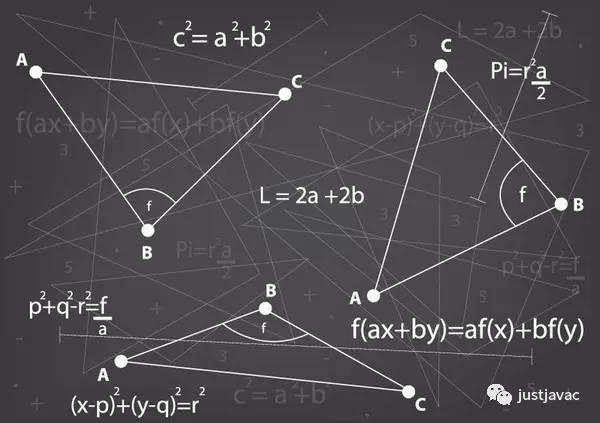Math.pow(99,99) 的结果是 3.697296376497263e+197，

3.697296376497263e+197
3.697296376497268e+197

``````function diff(x) {
return Math.pow(x,x) - x**x;
}
``````

``````var x = 99;
x**x - 99**99;
``````

``````for (let i = 0; i < 100*100*100; i++){
// 循环体
}
``````

``````for (let i = 0; i < 1000000; i++){
// 循环体
}``````

``````case Token::EXP: {
double value = Pow(x_val, y_val);
int int_value = static_cast<int>(value);
*x = factory()->NewNumberLiteral(
int_value == value && value != -0.0 ? int_value : value, pos,
has_dot);
return true;``````

``````// ES6 section 20.2.2.26 Math.pow ( x, y )
TF_BUILTIN(MathPow, CodeStubAssembler) {
Node* x = Parameter(1);
Node* y = Parameter(2);
Node* context = Parameter(5);
Node* x_value = TruncateTaggedToFloat64(context, x);
Node* y_value = TruncateTaggedToFloat64(context, y);
Node* value = Float64Pow(x_value, y_value);
Node* result = ChangeFloat64ToTagged(value);
Return(result);
}``````

``````Expression* Parser::RewriteExponentiation(
Expression* left,
Expression* right,
int pos) {
ZoneList<Expression*>* args = new (zone()) ZoneList<Expression*>(2, zone());
return factory()->NewCallRuntime(Context::MATH_POW_INDEX, args, pos);
}``````

``````console.log(99**99);
a = 99, b = 99;
console.log(a**b);
console.log(Math.pow(99, 99));
``````

3.697296376497268e+197
3.697296376497263e+197
3.697296376497263e+197

9999=369729637649726772657187905628805440595668764281741102430259972423552570455277523421410650010128232727940978889548326540119429996769494359451621570193644014418071060667659301384999779999159200499899

--------------------------